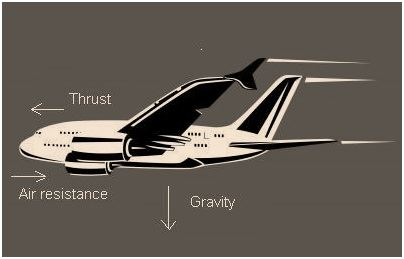# Air Resistance Formula

As we understand, the air resistance decreases the speed of a plane while moving.Air resistance is a force that is caused due to air when an object moves through it. This force acts in the opposite direction to a body passing through the air. Air resistance exerts a frictional force against the moving body. As a body moves, air resistance decelerates it down. The quicker the body’s motion, the superior the air resistance applied against it. Air resistance impacts all moving objects like bicycles, car, trains, rockets, aeroplanes and even alive bodies.

Its formula is given as,

Where,

F is the force of air resistance
c is the force constant

v is the object’s velocity

The negative sign indicates that the direction of air resistance is opposite to the direction of motion of the object.

Air Resistance Formula is made use of in finding the air resistance, air constant and velocity of the body if some of these numerics are known. This formula has wide applications in aeronautics.

## Air Resistance Solved Examples

Problem 1: A plane moving with a velocity of 50 ms-1 has a force constant of 0.05. Calculate its air resistance.

Given:

Velocity of air, v = 40 ms-1,
Force constant, c = 0.05
The Force of air is given by F = – cv2 = 0.05 × 1600 = – 80 N

Problem 2: An object is travelling at a speed of 20 ms-1. The object experiences a force of 50 N. Calculate the force constant.